TitleCollege Algebra
Answer/Discussion to Practice Problems
Tutorial 13: Practice Test on Tutorials 2 - 12

 Problems 1a - 1d:  Simplify the exponential expression.

 1a.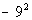Answer: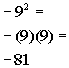1b.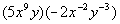Answer: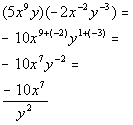1c.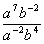Answer: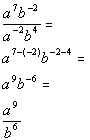1d.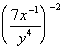Answer: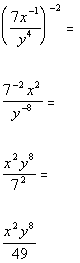Problem 2a:  Write the number in scientific notation.

 2a.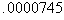Answer: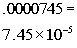Problem 3a:  Write the number in decimal notation, without exponents.

 3a.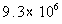Answer: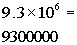Problems 4a - 4b:  Evaluate.

 4a.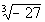Answer: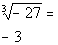4b.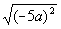Answer: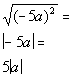Problems 5a - 5b:  Simplify.

 5a.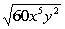Answer: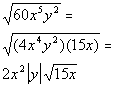5b.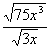Answer: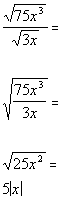Problem 6a:  Perform the indicated operation.

 6a.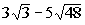Answer: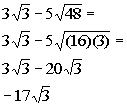Problems 7a - 7b:  Rationalize the denominator.

 7a.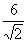Answer: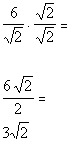7b.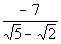Answer: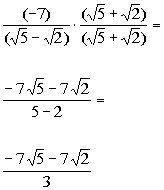Problems 8a - 8c:  Simplify.

 8a.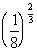Answer: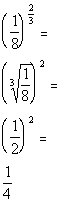8b.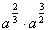Answer: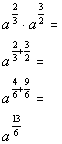8c.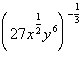Answer: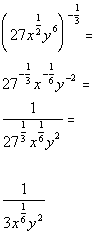Problems 9a - 9c:  Perform the indicated operation.

 9a.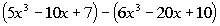Answer: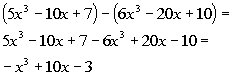9b.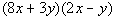Answer: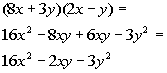9c.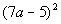Answer: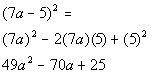Problems 10a - 10d:  Factor completely.

 10a.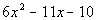Answer: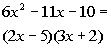10b.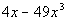Answer: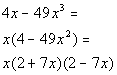10c.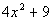Answer: Prime.

 10d.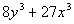Answer: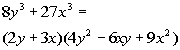Problems 11a - 11c:  Perform the indicated operation.

 11a.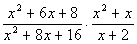Answer: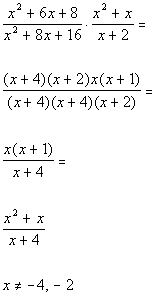11b.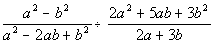Answer: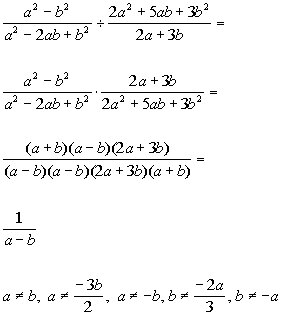11c.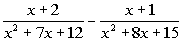Answer: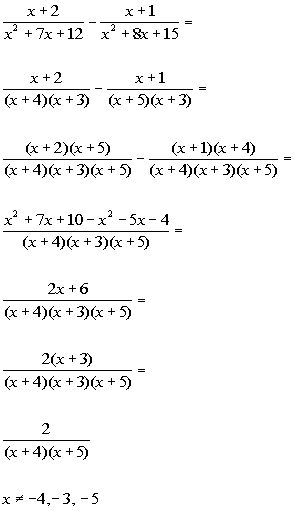Problem 12a:  Simplify.

 12a.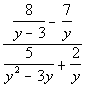Answer: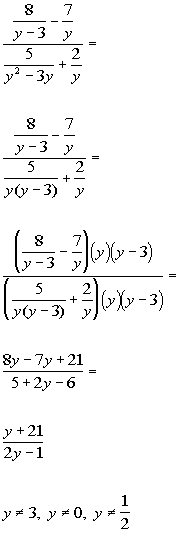Problems 13a - 13e:  Perform the indicated operation.  Write the answer in standard from.

 13a.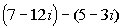Answer: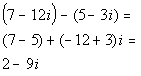13b.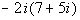Answer: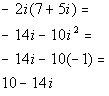13c.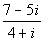Answer: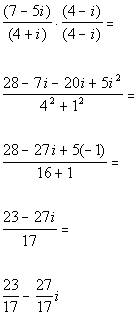13d.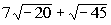Answer: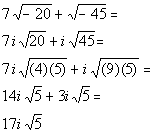13e.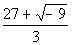Answer: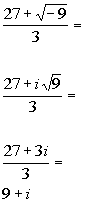Last revised on Dec. 15, 2009 by Kim Seward.
All contents copyright (C) 2002 - 2010, WTAMU and Kim Seward. All rights reserved.